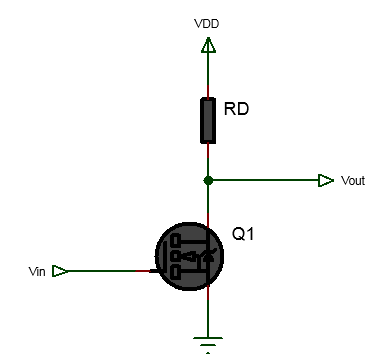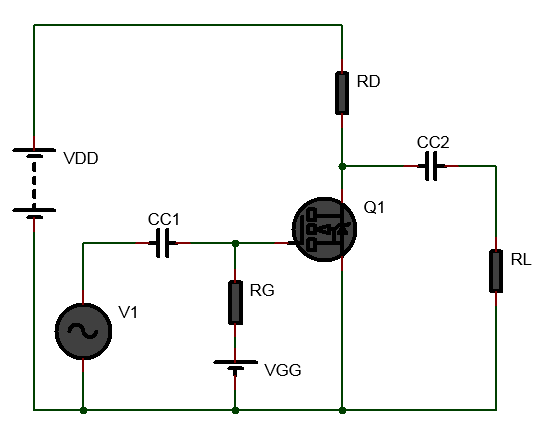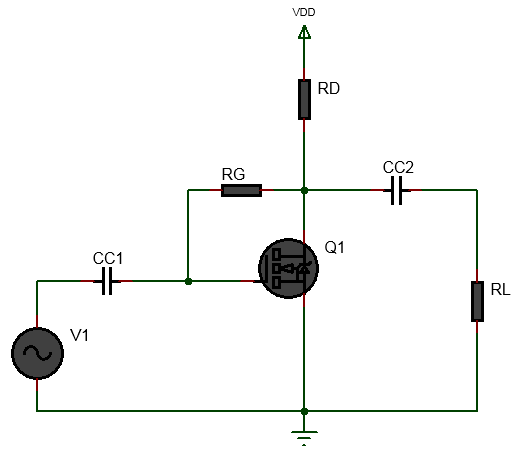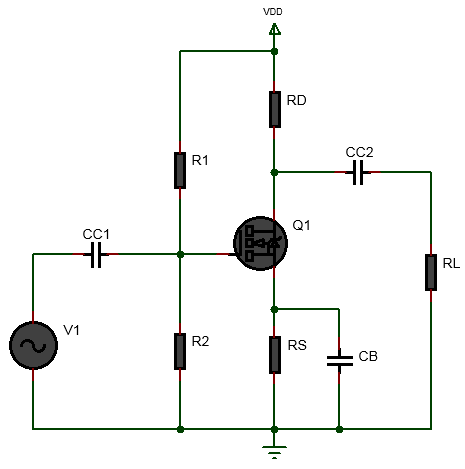# E-MOSFET biased in Ohmic Region

Inputs:
V
V
V
mA
mA

Theoritical Results:### Enhancement MOSFET Biased in Ohmic Region

Enhancement MOSFET are used usually used as switches. When E-MOSFET are operated as switches they are biased in the Ohmic region. To bias E-MOSFET in the ohmic region, the saturation drain current($$I_{D(sat)}$$) must be less than the ON drain current($$I_{D(sat)}$$) when gate to souce voltage($$V_{GS}$$) is equal to the ON gate to souce voltage($$V_{GS(ON)}$$). In this condition, the E-MOSFET acts as a resistor with resistance of $$R_{DS(on)} = \frac{V_{DS(on)}}{R_{DS(on)}}$$.
A tutorial on biasing enhancement MOSFET in the ohmic region to operate the MOSFET as a switch is provided in Biasing Enhancement MOSFET in Ohmic Region to operate as switch.

### Equations Used:

(1) Obtain, $$V_{GS} = V_{GS(on)}$$ $$\newline$$ (2) Obtain $$V_{th}$$ $$\newline$$ (3) Let $$V_{DS(on)}=V_{th}$$ $$\newline$$ (4) Determine $$I_{D(on)}$$ at $$V_{GS} = V_{GS(on)}$$ and $$V_{DS(on)}=V_{th}$$ $$\newline$$ (5) Let $$V_{DSQ} = \frac{V_{DS(on)}}{2}$$ $$\newline$$ (6) Find $$I_{DQ}$$ at $$V_{GS} = V_{GS(on)}$$ and $$V_{DSQ}$$ $$\newline$$ (7) Determine $$R_D$$,
$$R_D = \frac{V_{DD}-V_{DSQ}}{I_{DQ}}$$ $$\newline$$ (8) Find $$R_{DS(on)}=\frac{V_{DS(on)}}{I_{D(on)}}$$

# Enhancement MOSFET Fixed Gate Bias Calculator

Inputs:

V
V
V
mA
V

Theoritical Results:## Fixed Gate Biased Enhancement MOSFET Amplifier and Biasing Calculator

The above Enhancement MOSFET fixed gate bias and amplifier design calculator helps to calculate the drain resistor($$R_D$$) value, the coupling capacitors values, the voltage gain, the transconductance value from given gate to source voltage($$V_{GS}$$), the drain current($$I_D$$), the gate to source threshold voltage($$V_{GS(th)}$$), the drain voltage($$V_D$$) which is equal to the drain to source voltage( ($$V_{DS}$$)) and the frequency of the input signal.

In fixed gate biasing method of E-MOSFET, a positive gate to source voltage is applied to the gate which sets up gate to source voltage that then set ups the drain current and voltage. When positive gate voltage is applied then the MOSFET operates in enhancement mode. The fixed gate bias method when applied to E-MOSFET will bias the E-MOSFET in its active region. When E-MOSFET is biased in active region it can be used as an amplifier.

The tutorial Fixed Gate Biased Enhancement MOSFET Amplifier explains step by step the process of biasing a depletion MOSFET with gate bias(fixed bias).

### Equations Used:

First obtain $$I_{DSS}$$ and $$V_{GS(off)}$$ from the MOSFET datasheet. $$\newline$$ (1) $$V_{GS} = V_{GS(off)}(1 - \sqrt{\frac{I_D}{I_{DSS}}})$$ $$\newline$$ (2) $$R_D=\frac{V_{DD}-V_{DS}}{I_D}$$ $$\newline$$ (3) $$Z_i = R_G$$ $$\newline$$ (4) $$Z_o = R_D$$ $$\newline$$ (5) $$CC_1 = \frac{10}{2\pi f Z_i}$$ $$\newline$$ (6) $$CC_2 = \frac{10}{2\pi f Z_o}$$

# Drain Feedback Biased E-MOSFET Amplifier Calculator

Inputs:

V
V
V

V
V

Theoritical Results:## Drain Feedback Biased E-MOSFET Amplifier Design Calculator

This Drain Feedback Biased E-MOSFET Amplifier Design Calculator helps you to bias an enhancement MOSFET using drain feedback biasing method to design E-MOSFET amplifier. That is it helps to calculate the drain resistor value, helps to calculate the transconductance value, voltage gain, Furthermore, it helps to calculate the input and output impedances and the coupling capacitors and bypass capacitor values.

In drain feedback biased enhancement MOSFET, a feedback resistor is connected from drain back to the gate. This is done to compensate any change in the drain current and voltage. For example, consider that due to temperature increase, the drain current is increased. This increase in drain current decreases the drain voltage or the drain to souce voltage(since there is no resistor at the source). The decrease in the drain voltage in turn causes the gate to source voltage to decrease. This is because in case of drain feedback bias, the gate to source voltage(the gate voltage) is equal to the drain voltage(or the drain to source voltage). And because the gate to source voltage is decreased the drain current is decreased. So while initially the drain current increased due to increase in temperature, at the end the drain current is decreased due to the feedback effect. Same effect happens if the drain current is decreased(due to feedback effect, the drain current is increased). So any increase or decrease of the drain current is compensated by the feedback bias circuit.

For example of using this calculator see E-MOSFET Amplifier: Solution with Drain Feedback Bias.A detailed worked out example is shown in the tutorial Drain Feedback Biased Enhancement MOSFET.

### Equations Used:

(1) Find $$V_{GS(on)}$$,$$I_{D(on)}$$,$$V_{GS(th)}$$ from the datasheet $$\newline$$ (2) Pick Q-point $$V_{GSQ}$$ which must be greater than $$V_{GS(th)}$$ $$\newline$$ (3) Pick $$V_D$$ (for drain feedback bias it is equal to $$V_{DS}$$) $$\newline$$ (4) Determine $$k=\frac{I_{D(on)}}{(V_{GS(on)}-V_{GS(th)})^2}$$ $$\newline$$ (5) Find $$I_{DQ}=k[(V_{GSQ}-V_{GS(th)})^2]$$ $$\newline$$ (6) Find, $$R_D = \frac{V_{DD}-V_D}{I_{DQ}}$$ $$\newline$$ (7) $$R_d = R_D||R_L=\frac{R_D R_L}{R_D+R_L}$$ $$\newline$$ (8) $$g_m=2k(V_{GS}-V_{GS(th)})$$ $$\newline$$ (9) $$A_v = (-)g_m R_d$$ $$\newline$$ (10) $$Z_i = \frac{R_G}{1+g_m R_d}$$ [assuming $$r_d >10R_d$$] $$\newline$$ (11) $$Z_o = R_d \space [Z_o=r_d||R_d\space assuming \space r_d >> R_d]$$ $$\newline$$ (12) $$CC_1 = \frac{10}{2\pi f Z_i}$$ $$\newline$$ (13) $$CC_2 = \frac{10}{2\pi f Z_o}$$ $$\newline$$

# Voltage Divider Biased E-MOSFET Amplifier Calculator

Inputs:

V
V
V

V
V
V

Theoritical Results:## Voltage divider biased enhancement MOSFET amplifier

The Voltage divider biased enhancement MOSFET amplifier calculator above helps to calculate the biasing resistor values and coupling and by-pass capacitor values for given input specification. This is helpful in quick determination for components values for designing Voltage divider biased Depletion MOSFET amplifier. Voltage divider biasing is best suited for stable operation among the biasing methods. For detailed steps and explanations see Enhancement MOSFET Voltage Divider Biasing

### Equations Used:

(1) Find $$V_{GS(th)}$$, $$V_{GS}$$,$$V_{DS}$$ and $$I_D$$ for Q-point from datasheet or drain curve $$\newline$$ (2) Assume, $$V_D$$ $$\newline$$ (3) Calculate, $$V_{S} = V_D-V_{DS}$$ $$\newline$$ (4) Calculate, $$V_G=V_{GS}+V_S$$ $$\newline$$ (5) Calculate, $$R_D = \frac{V_{DD}-V_D}{I_D}$$ $$\newline$$ (6) Calculate,$$R_S = \frac{V_S}{I_D}$$ $$\newline$$ (7) Calculate, $$R_{1}=R_2(\frac{V_{DD}}{V_{G}}-1) \space [assume \space R_2]$$ $$\newline$$ (9) Calculate, $$R_d = R_D || R_L = \frac{R_D R_L}{R_D+R_L}$$ $$\newline$$ (10) Calculate, $$k = \frac{I_D}{(V_{GS} - V_{GS(th)})^2}$$ $$\newline$$ (11) $$g_m = 2k(V_{GS} - V_{GS(th)})$$ $$\newline$$ (12) $$A_v = g_m R_d$$ $$\newline$$ (13) $$Z_i = R_1 || R_2$$ $$\newline$$ (14) $$Z_o = R_d$$ $$\newline$$ (15) $$CC_1 = \frac{10}{2\pi f Z_i}$$ $$\newline$$ (16) $$CC_2 = \frac{10}{2\pi f Z_o}$$ $$\newline$$ (17) $$CB = \frac{10}{2\pi f R_S}$$ $$\newline$$